# ISEVEN in ExcelArticle byExcelMojo Team## What Is ISEVEN Function In Excel?

The ISEVEN function in Excel identifies the even numbers and returns TRUE if found, else returns FALSE. The function detects an even number as a cell value or the result of a formula while performing mathematical calculations.

The ISEVEN Excel function is an inbuilt Information function, so we can insert the formula from the “Function Library” or enter it directly in the worksheet.

For example, we will determine if the numbers are even values and display the result as logical values, TRUE or FALSE.

The table below contains a list of numbers.

Select cell B2, enter the formula =ISEVEN(A2), press “Enter”, and drag the formula from cell B2 to B7 using the fill handle. The output is shown below. Column C is for our reference.

[Output Observation: For instance, the cell B2 formula checks if the cell A2 value, 100, is even, and returns TRUE.

In the case of the cell B6 formula, the specified value, 2.253, gets truncated to 2. And as the truncated value is even, the function returns TRUE as the output.]

###### Key Takeaways
• The ISEVEN function in Excel determines if the specified number is even. It always returns a logical value as output, with its return value being TRUE if the given value is even. And the function output is FALSE if the specified number is odd.
• We can use the function with other Excel functions such as IF(), and SUM() and also use the Conditional Formatting method.
• Use the method Application.WorksheetFunction.ISEVEN(Arg1) to implement the function ISEVEN in Excel VBA. The Arg1 argument represents the number argument in the ISEVEN().

### ISEVEN() Excel Formula

The syntax of the ISEVEN Excel formula is,

The argument of the ISEVEN Excel formula is,

• number: The dataset to test whether the numeric values are even. It is a mandatory argument.

### How To Use ISEVEN Excel Function?

We can use the ISEVEN function in 2 ways, namely,

1. Access from the Excel ribbon.
2. Enter in the worksheet manually.

#### Method #1 – Access from the Excel ribbon

Choose an empty cell for the output → select the “Formulas” tab → go to the “Function Library” group → click the “More Functions” options drop-down → click the “Information” option right arrow → select the “ISEVEN” function, as shown below.

The “Function Arguments” window appears. Enter the argument in the “Number” field → click “OK”, as shown below.

#### Method #2 – Enter in the worksheet manually

1. Select an empty cell for the output.
2. Type =ISEVEN( in the cell. [Alternatively, type =I or =IS and double-click the ISEVEN function from the Excel suggestions.]
3. Enter the arguments as cell values or cell references and close the brackets.
4. Press Enter to view the outcome.

Let us take a basic example to understand this function.

We will determine if the dataset contains even values using ISEVEN function.

The table below shows a list of data values and their descriptions.

The steps to find the even numbers using ISEVEN() are,

1. Select cell C2, and enter the formula =ISEVEN(A2).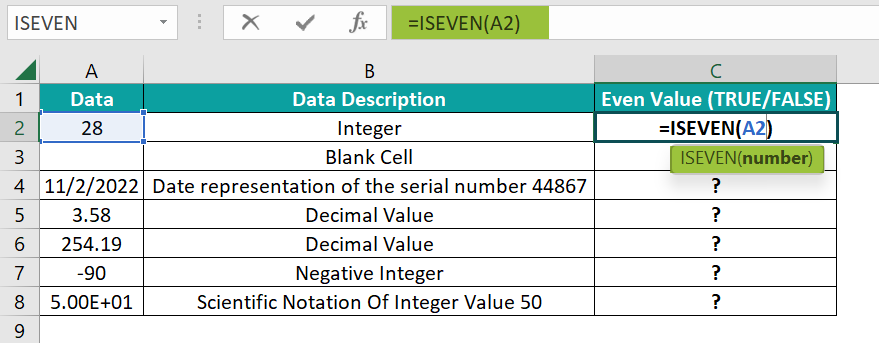2. Press the Enter. The result is “TRUE”, as shown below.

[Note: We can use alternate formulas such as =ISEVEN(28) or =ISEVEN(“28”) in the target cells to get the same results.]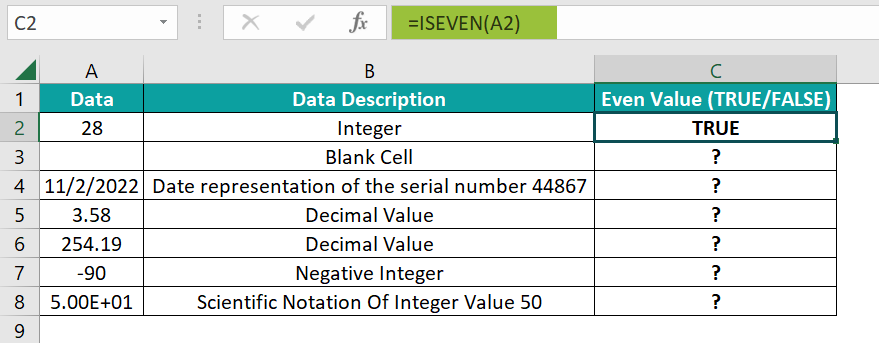3. Drag the formula from cell C2 to C8 using the fill handle. The output is shown below.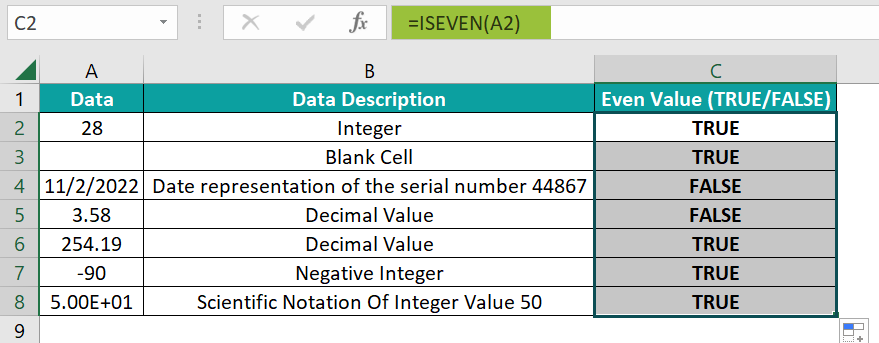[Alternatively, select the target cell C2 and click Formulas More FunctionsInformationISEVEN to access the function from the Formulas tab.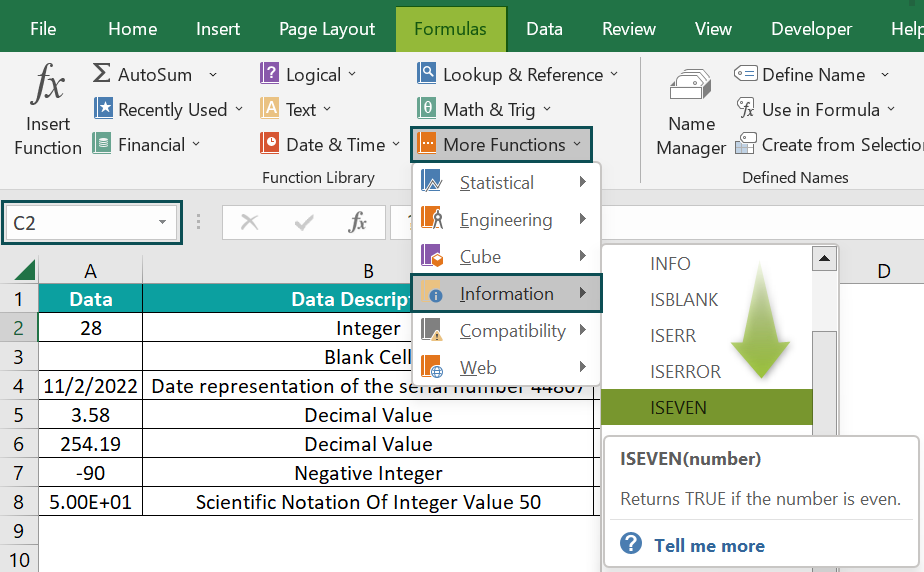Following the above step opens the Function Arguments window, where we enter the specified number or cell reference to the number. Now, click OK to close the Function Arguments window, and we will see the ISEVEN() output in cell C2.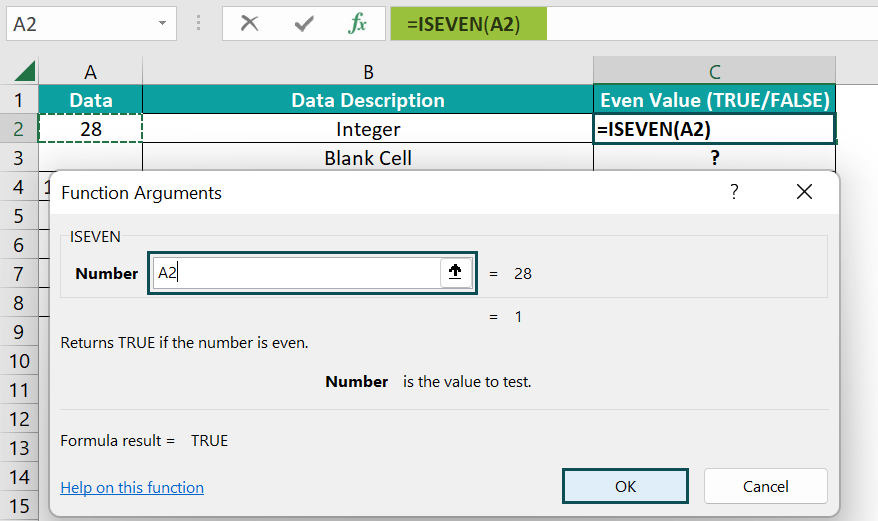[Output Observation: In row 2, the specified value is an integer. And as it is even, the function output is TRUE. In the case of row 3, the specified argument value is an empty cell. The function evaluates it as 0 and returns TRUE as the outcome.

Cell A4 contains a date, which represents the serial number 44867. And as the serial number is odd, the function return value is FALSE.

On the other hand, cells A5:A6 contain decimals. So, the function in the corresponding target cells C5 and C6 truncate the decimal values to 3 and 254, respectively. And as the truncated values are odd and even, the function outputs in the corresponding target cells are FALSE and TRUE, respectively.

In the case of row 7, the specified value is a negative integer. However, it is even. And hence, the function output is TRUE.

Finally, cell A8 contains the scientific notation of the integer 50. Again, as the integer 50 is even, the function returns TRUE as the output.]

### Examples

We will understand some advanced scenarios using the ISEVEN function examples.

#### Example #1

We will determine if packing the total stock (in each row) in units of 2 is feasible, and summing the day-wise stock in each row using the and use the ISEVEN function.

The below table contains the five days’ stock details of five items.

The steps to find the even numbers using ISEVEN() and SUM() functions are,

• Step 1: Select the target cell G2, enter the formula =ISEVEN(SUM(B2:F2)), and press Enter. The result is ‘False’, as shown below.
• Step 2: Drag the formula from cell G2 to G6 using the fill handle. The output is shown below.

[Output Observation: In cell G1, first, the SUM excel function returns the day 1 to day 5 stock total of 153. Next, the ISEVEN function checks whether the total stock value is even. And as 153 is odd, the ISEVEN() returns FALSE as the status.

In the case of row 5, the total stock is 0, and as it is even, the ISEVEN function returns TRUE in cell G5.]

#### Example #2

We must split the employees into teams A and B, according to the employee IDs using the ISEVEEN() and the IF Excel functions.

The below table contains a list of employees and their employee IDs.

The steps to find the even numbers using ISEVEN() and IF() functions are,

• Step 1: Select cell C2, enter the formula =IF(ISEVEN(A2),”Team A”,”Team B”), and press Enter. The result is “Team A”, as shown below.
• Step 2: Drag the formula from cell C2 to C11 using the fill handle. The output is shown below.

[Output Observation: In cell C11, first, the ISEVEN function checks if the specified employee ID, 1739, is even. And as the value is odd, the function returns FALSE.

Next, as the IF condition is FALSE, it returns the FALSE value, Team B, as the allotted team for the employee, John Adams.]

#### Example #3

In the given dataset, we have two categories, with each category in alternate cells. We will highlight the alternate rows of data to show the categories distinctively using the ISEVEN() and Conditional Formatting.

The following table shows US’s leading laptop and smartphone models.

The steps to find the even numbers using ISEVEN() and Conditional Formatting are,

• Step 1: Select the cell range A3:B12 and go to Home Conditional Formatting New Rule.

The New Formatting Rule window opens, as shown below.

• Step 2: In the “Select a Rule Type:” group, choose the “Use a formula to determine which cells to format” option, as shown below.
• Step 3: Now, in the “Edit the Rule Description:” group, enter the formula =ISEVEN(ROW(A3)), and click the “Format” button, as shown below.

This step will open the Format Cells window, as shown below.

• Step 4: Now, go to the Fill tab, pick a color to highlight the qualifying row cells, and click OK to close the Format Cells window.
• Step 5: Click OK in the New Formatting Rule window to close it and view the highlighted rows in the source table.

We will get the following output.

[Output Observation: First, the ROW excel function returns the row number of the specified cell. And then, the ISEVEN function checks if the row number the ROW() returns is even.

As the conditional formatting rule applies to the cell range A3:B12, each cell gets checked for an even row number. And those cells for which the ISEVEN function returns TRUE, the cell gets highlighted in the chosen color.]

### Important Things To Note

• The ISEVEN function argument value can be the specified number, with or without the double quotations, or the cell reference to the number in question.
• For a non-numeric argument value, the ISEVEN function throws the #VALUE! error.
• If the specified value is a fraction, Excel converts it into a decimal, truncates it, checks if the truncated value is even, and returns the appropriate logical value.

1. Is there an ISEVEN function in Excel?

There is an ISEVEN function in Excel available in the Formulas tab.

Select a cell, go to FormulasMore FunctionsInformationISEVEN, as shown below.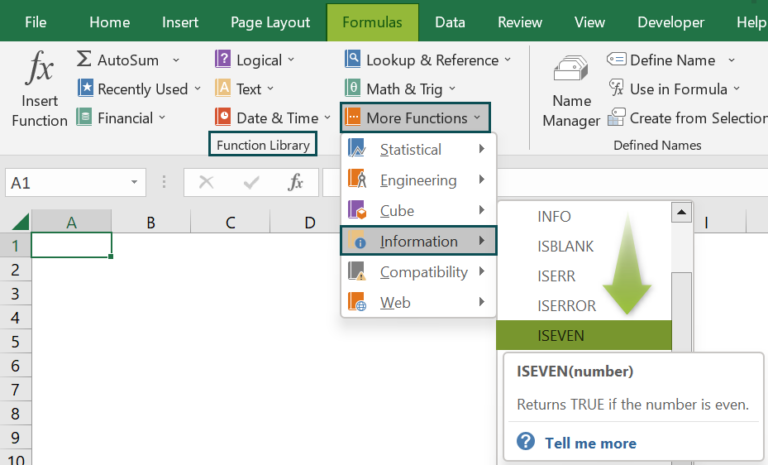2. How to apply the ISEVEN function in Excel VBA?

We can apply the ISEVEN function in Excel VBA using the method:

Application.WorksheetFunction.ISEVEN(Arg1)

For instance, we will find whether the listed numbers are even, using the ISEVEN and the Excel VBA.

The following table contains a list of numbers.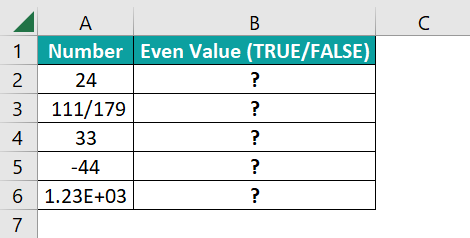The steps to find the even numbers using the ISEVEN in Excel VBA are,

• Step 1: Open the worksheet containing the above table and press Alt + F11 to open the VBA Editor.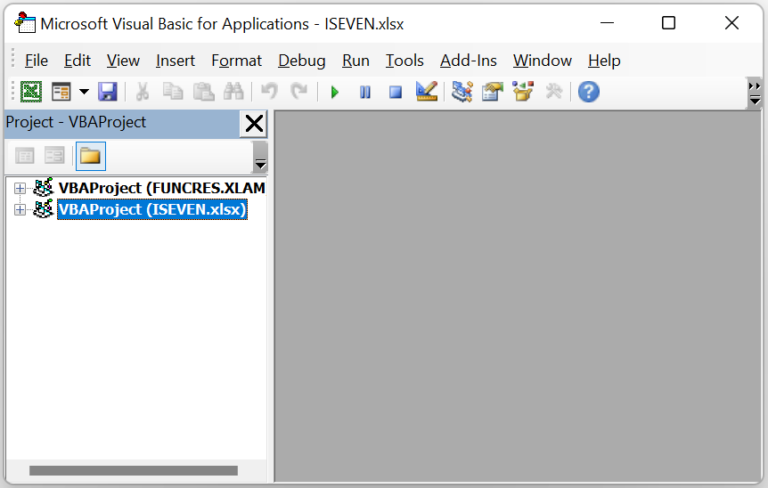• Step 2: Pick the applicable VBAProject and choose the Module option from the Insert tab. This action will open a new module window, Module1.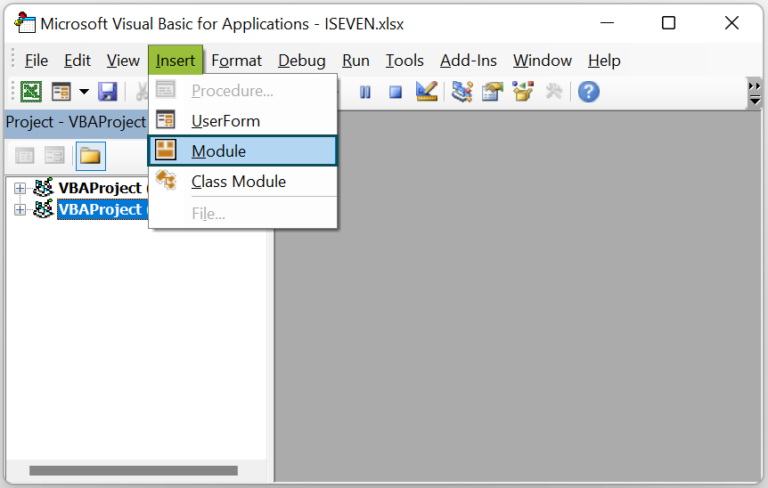• Step 3: Enter the VBA code, shown below, to apply the ISEVEN() in the target cells.

Sub ISEVEN_fn()

Dim ws As Worksheet
Set ws = Worksheets(“FAQ_2”)

ws.Range(“B2”) = Application.WorksheetFunction.IsEven(ws.Range(“A2”))
ws.Range(“B3”) = Application.WorksheetFunction.IsEven(ws.Range(“A3”))
ws.Range(“B4”) = Application.WorksheetFunction.IsEven(ws.Range(“A4”))
ws.Range(“B5”) = Application.WorksheetFunction.IsEven(ws.Range(“A5”))
ws.Range(“B6”) = Application.WorksheetFunction.IsEven(ws.Range(“A6”))

End Sub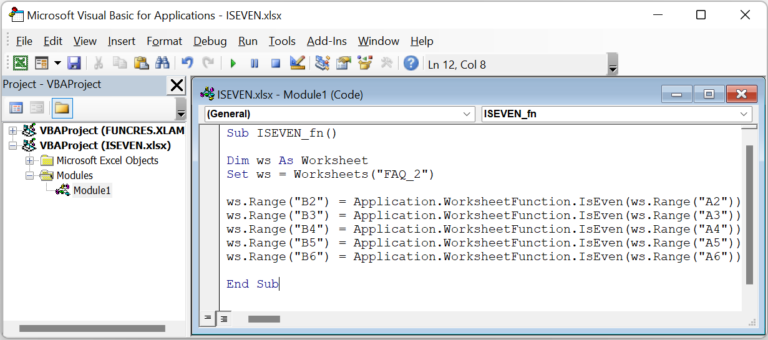• Step 4: Click the Run Sub/UserForm button from the menu to run the code.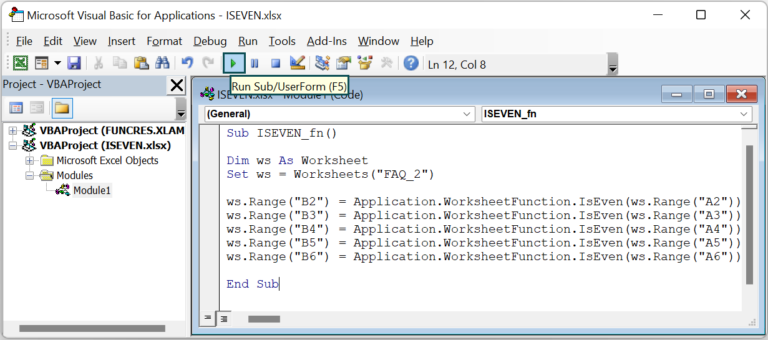Finally, open the active worksheet to view the result in the target cells.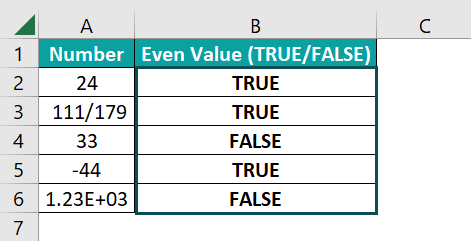[Output Observation: Cells A2 and A5 values are even numbers, the ISEVEN() in the respective target cells show TRUE as the output. In the case of cell B3, the supplied fractional number is the equivalent of the decimal value 0.620111. And as the function truncates the decimal value to 0, which is an even number, it returns TRUE as the output.
On the other hand, the cell A4 value is odd. So, the corresponding target cell B4 shows the output as FALSE. And as cell C6 contains the scientific notation of the number 1231, an odd value, the function output is FALSE.]

3. Why is the ISEVEN function not working in Excel?

The ISEVEN may not work due to the following reasons.
The supplied argument value is non-numeric.
We executed the function as an array formula.